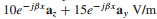### Create an Account

Already have account?

### Forgot Your Password ?

Home / Questions / A uniform plane wave in free space has electric field vector given by Es a Describe the ...

# A uniform plane wave in free space has electric field vector given by Es a Describe the wave polarization b Find Hs c Determine the average power density in the wave in W/m2 2 Consider a

A uniform plane wave in free space has electric field vector given by Es =(a) Describe the wave polarization. (b) Find Hs. (c) Determine the average power density in the wave in W/m2.

2. Consider a left circularly polarized wave in free space that propagates in the forward z direction. The electric field is given by the appropriate form of Eq. (100). Determine (a) the magnetic field phasor, Hs; (b) an expression for the average power density in the wave in W/m2 by direct application of Eq. (77)

Jun 13 2020 View more View Less

#### Answer (Solved)Subscribe To Get Solution